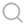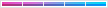• 622阅读
• 0回复

# [移动开发]ES6之箭头函数40

## 语法

``````([param] [, param]) => {
statements
}

param => expression ```

1

2

3

4

5

6

```

param 是参数，根据参数个数不同，分这几种情况：
() => { … } // 零个参数用 () 表示；
x => { … } // 一个参数可以省略 ()；
(x, y) => { … } // 多参数不能省略 ()；

## 示例

``````// ES5 var selected = allJobs.filter(function (job) { return job.isSelected();
}); // ES6 var selected = allJobs.filter(job => job.isSelected()); ```

1

2

3

4

5

6

7

8

```

``````// ES5 var total = values.reduce(function (a, b) { return a + b;
}, 0); // ES6 var total = values.reduce((a, b) => a + b, 0); ```

1

2

3

4

5

6

7

8

```

``````// ES5 \$("#confetti-btn").click(function (event) { playTrumpet();
fireConfettiCannon();
}); // ES6 \$("#confetti-btn").click(event => {
playTrumpet();
fireConfettiCannon();
}); ```

1

2

3

4

5

6

7

8

9

10

11

```

````x => { return x * x }; // 函数返回 x * x x => x * x; // 同上一行 x => return x * x; // SyntaxError 报错，不能省略 {} x => { x * x }; // 合法，没有定义返回值，返回 undefined `

1

2

3

4

5

```

``````var func1 = (x = 1, y = 2) => x + y;
func1(); // 得到 3 var func2 = (x, ...args) => { console.log(args) };
func2(1,2,3); // 输出 [2, 3] ```

1

2

3

4

5

6

```

## 特性

``````var o = {
x : 1,
func : function() { console.log(this.x) },
test : function() { setTimeout(function() { this.func();
}, 100);
}
};

o.test(); // TypeError : this.func is not a function ```

1

2

3

4

5

6

7

8

9

10

11

12

```

``````var o = {
x : 1,
func : function() { console.log(this.x) },
test : function() { var _this = this;
setTimeout(function() { _this.func();
}, 100);
}
};

o.test(); ```

1

2

3

4

5

6

7

8

9

10

11

12

13

```

``````var o = {
x : 1,
func : function() { console.log(this.x) },
test : function() {
setTimeout(() => { this.func() }, 100);
}
}; o.test(); ```

1

2

3

4

5

6

7

8

9

10

```

``````var x = 1,
o = {
x : 10,
test : () => this.x
};

o.test(); // 1 o.test.call(o); // 依然是1``````您目前还是游客，请 登录 或 注册